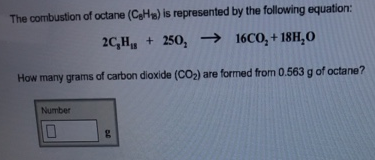# Problem: The combustion of octane (C8H18) is represented by the following equation: 2C8H18 + 25O2 → 16CO2 + 18H2O How many grams of carbon dioxide (CO2) are formed from 0.563 g of octane?

###### FREE Expert Solution
90% (235 ratings)###### Problem Details

The combustion of octane (C8H18) is represented by the following equation: 2C8H18 + 25O2 → 16CO2 + 18H2O How many grams of carbon dioxide (CO2) are formed from 0.563 g of octane?